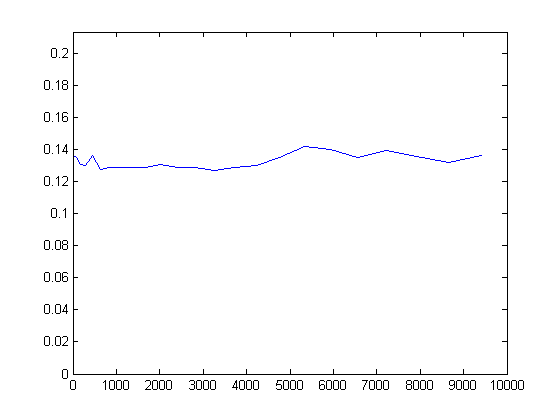# Dilation algorithms—Introduction

Post updated on September 4 based on feedback from Cris Luengo's comment.

Today I'm starting a new series covering some of the concepts underlying algorithms for performing morphological dilation (and erosion, which is very similar). Most of the time, when people talk about image dilation, they mean the form of dilation that is a local maximum operation on the neighbors of each pixel. For example, here's how to compute the local maximum, for each image pixel, with that pixel and its eight neighbors:

A = magic(5)
A =

17    24     1     8    15
23     5     7    14    16
4     6    13    20    22
10    12    19    21     3
11    18    25     2     9


se = ones(3,3)
se =

1     1     1
1     1     1
1     1     1


B = imdilate(A, se)
B =

24    24    24    16    16
24    24    24    22    22
23    23    21    22    22
18    25    25    25    22
18    25    25    25    21



The set of neighborhood pixels involved in the max operator forms a shape called the structuring element. The structuring element doesn't have to be square, as above. Here is dilation with a short, 3-pixel line:

se = [1 1 1];
B2 = imdilate(A, se)
B2 =

24    24    24    15    15
23    23    14    16    16
6    13    20    22    22
12    19    21    21    21
18    25    25    25     9



Let's consider how long it should take to compute dilation. Suppose an image has P pixels, and the structuring element contains Q neighbors. Computing the output for a particular pixel involves both Q memory reads and Q comparisons. Therefore we might expect the computation time to be proportional to Q.

We can try to verify that behavior using square structuring elements of varying sizes.

% Read in a small sample image and replicate it to make a 1k-by-1k
% test image.

% Measure dilation times using a set of square structuring elements
% ranging in size from 5-by-5 to 97-by-97.
w = 5:4:97;

% The number of neighbors, Q, in each structuring element will be
% w^2.  We are guessing that the running time will be proportional
% to Q.
Q = w.^2;

% Initialize vector containing recorded times.
times = [];

for wk = w
% Not that many MATLAB users are familiar with the for-loop
% construct above.  I'll send a MATLAB t-shirt to the first
% person who adds a comment to this post with a brief, clear,
% and correct explanation.

se = strel(ones(wk, wk));
f = @() imdilate(I, se);
times(end+1) = timeit(f);
end

plot(Q, times)
ylim([0 1.5*max(times)])That plot looks almost constant, with maybe just a hint of an increase with Q.

So is imdilate somehow computing dilation faster than expected?

That's the subject of this series. We'll start digging in deeper next time.

Published with MATLAB® 7.6

|